Physic Tutorials

# Notes on wave motion for Cambridge A level and UTME

Notes on wave motion for Cambridge A level and UTME

A wave allows energy to be transferred from one point to another without any particle of the medium travelling between the two points.

Wave motion can be classified base on:

• Mode of Propagation

The class of waves under this are

Mechanical wave: this requires a material medium for propagation

Electromagnetic wave: This travels in a vacuum

• Mode of vibration

The class of waves under this are:

(i) Longitudinal waves

(ii) Transverse waves

Diagramatic representation of waves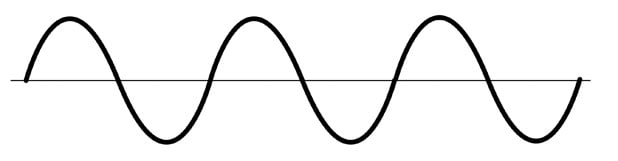Definition of terms

Period (T): it is the time taken for a particle to undergo one complete cycle of oscillation.

Frequency (f): it is the number of oscillations performed by a particle per unit time.

Wavelength (λ): it is the distance between any two successive particles that are in phase, e.g. it is the distance between 2 consecutive crests or 2 troughs.

Wave speed (v): The speed at which the waveform travels in the direction of the propagation of the wave.

Wave front: A line or surface joining points which are at the same state of oscillation, i.e. in phase, e.g. a line joining crest to crest in a wave.

Displacement : it is the Position of an oscillating particle from its equilibrium position.

Amplitude: it is the maximum magnitude of the displacement of an oscillating particle from its equilibrium position.

To deduce V = fλ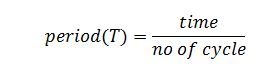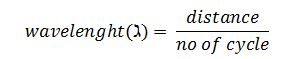no of cycle = n

time = Tn

distance = λn

substitute all into the velocity = distance/timeNoteF is the frequency and the S.I base unit is Hertz(Hz)

T is the period and the S.I base unit is second(s)

λ is the wavelength and the S.I base unit is meter(m)

therefore,

V = fλ

Displacement-distance graph and Displacement-time graph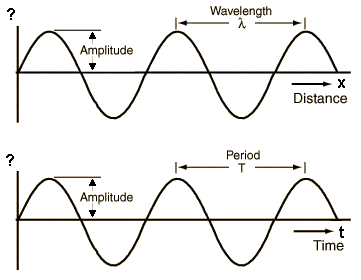The first graph is a displacement-distance graph. On this graph you can find wavelenght and amplitude

The second graph is a displacement-time graph. On this graph you can find period, frquency and amplitude

The only difference between the two graphs is what you can calculate from it.

Phase difference: this is an amount by which one oscillation leads or lags behiod another. it measures in degree or radian.

Phase difference between waves that are exactly out of phase is π radians or 180 degrees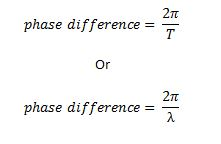progressive wave: it is a propagation of energy as a results of vibrations of waves which move energy from one place to another.

Intensity: it is defined as power incident per unit area. The intensity of wave generally decreases as it travels along. The two reasons for this are:

• The wave may spread out
• The wave may be absorbed or scattered

As wave spread out, its amplitude decreases

The unit of intensity is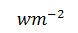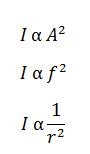I is the intensity

A is amplitude

f is frequency

r is the distance from the source

Difference between Longitudinal waves and Transverse waves

Transverse waves: A wave in which the oscillations of the wave particles are perpendicular to the direction of the propagation of the wave. Light wave is an example of transverse waves

Longitudinal waves: A wave in which the oscillations of the wave particles are parallel to the direction of the propagation of the wave. Sound wave is an example of longitudinal wave

Transverse waves can be plane polarised while longitudinal waves cannot be plane polarised.

Electromagnetic waves travel with the same speed in space

the speed of electromagnetic wave is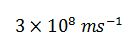Recommended: Solved questions on wave motion

Related Article: Short note on oscillation

If you enjoy this topic you can help me to share it with friends and love ones.

### Bolarinwa Olajire

A lecturer, Educationist, PhD student at FUNAAB, and a Blogger.